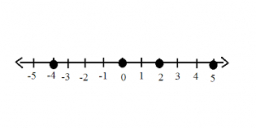# Find two 2

Find two decimals between 4.56 and 6.927 so that the difference between any two consecutive decimals is the same.

a =  5.349
b =  6.138

### Step-by-step explanation:Did you find an error or inaccuracy? Feel free to write us. Thank you!

Tips for related online calculators
Do you have a linear equation or system of equations and looking for its solution? Or do you have a quadratic equation?